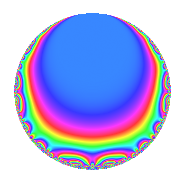# Properties

 Label 945.2.a.hLevel 945 Weight 2 Character orbit 945.a Self dual Yes Analytic conductor 7.546 Analytic rank 1 Dimension 2 CM No Inner twists 1

# Related objects

## Newspace parameters

 Level: $$N$$ = $$945 = 3^{3} \cdot 5 \cdot 7$$ Weight: $$k$$ = $$2$$ Character orbit: $$[\chi]$$ = 945.a (trivial)

## Newform invariants

 Self dual: Yes Analytic conductor: $$7.54586299101$$ Analytic rank: $$1$$ Dimension: $$2$$ Coefficient field: $$\Q(\sqrt{5})$$ Coefficient ring: $$\Z[a_1, a_2]$$ Coefficient ring index: $$1$$ Fricke sign: $$1$$ Sato-Tate group: $\mathrm{SU}(2)$

## $q$-expansion

Coefficients of the $$q$$-expansion are expressed in terms of $$\beta = \frac{1}{2}(1 + \sqrt{5})$$. We also show the integral $$q$$-expansion of the trace form.

 $$f(q)$$ $$=$$ $$q$$ $$+ \beta q^{2}$$ $$+ ( -1 + \beta ) q^{4}$$ $$- q^{5}$$ $$- q^{7}$$ $$+ ( 1 - 2 \beta ) q^{8}$$ $$+O(q^{10})$$ $$q$$ $$+ \beta q^{2}$$ $$+ ( -1 + \beta ) q^{4}$$ $$- q^{5}$$ $$- q^{7}$$ $$+ ( 1 - 2 \beta ) q^{8}$$ $$- \beta q^{10}$$ $$+ ( 2 - 2 \beta ) q^{11}$$ $$-4 \beta q^{13}$$ $$- \beta q^{14}$$ $$-3 \beta q^{16}$$ $$+ ( -3 + 2 \beta ) q^{17}$$ $$+ ( -5 + 4 \beta ) q^{19}$$ $$+ ( 1 - \beta ) q^{20}$$ $$-2 q^{22}$$ $$+ ( 5 - 2 \beta ) q^{23}$$ $$+ q^{25}$$ $$+ ( -4 - 4 \beta ) q^{26}$$ $$+ ( 1 - \beta ) q^{28}$$ $$+ ( 4 - 6 \beta ) q^{29}$$ $$-9 q^{31}$$ $$+ ( -5 + \beta ) q^{32}$$ $$+ ( 2 - \beta ) q^{34}$$ $$+ q^{35}$$ $$+ ( -6 + 6 \beta ) q^{37}$$ $$+ ( 4 - \beta ) q^{38}$$ $$+ ( -1 + 2 \beta ) q^{40}$$ $$+ ( -6 + 4 \beta ) q^{41}$$ $$+ ( -6 + 4 \beta ) q^{43}$$ $$+ ( -4 + 2 \beta ) q^{44}$$ $$+ ( -2 + 3 \beta ) q^{46}$$ $$+ 4 q^{47}$$ $$+ q^{49}$$ $$+ \beta q^{50}$$ $$-4 q^{52}$$ $$+ ( -3 + 8 \beta ) q^{53}$$ $$+ ( -2 + 2 \beta ) q^{55}$$ $$+ ( -1 + 2 \beta ) q^{56}$$ $$+ ( -6 - 2 \beta ) q^{58}$$ $$+ ( -8 + 6 \beta ) q^{59}$$ $$+ ( -3 - 6 \beta ) q^{61}$$ $$-9 \beta q^{62}$$ $$+ ( 1 + 2 \beta ) q^{64}$$ $$+ 4 \beta q^{65}$$ $$+ ( 6 - 8 \beta ) q^{67}$$ $$+ ( 5 - 3 \beta ) q^{68}$$ $$+ \beta q^{70}$$ $$-2 q^{71}$$ $$+ ( -2 - 4 \beta ) q^{73}$$ $$+ 6 q^{74}$$ $$+ ( 9 - 5 \beta ) q^{76}$$ $$+ ( -2 + 2 \beta ) q^{77}$$ $$+ ( -11 + 10 \beta ) q^{79}$$ $$+ 3 \beta q^{80}$$ $$+ ( 4 - 2 \beta ) q^{82}$$ $$+ 11 q^{83}$$ $$+ ( 3 - 2 \beta ) q^{85}$$ $$+ ( 4 - 2 \beta ) q^{86}$$ $$+ ( 6 - 2 \beta ) q^{88}$$ $$+ ( 2 - 10 \beta ) q^{89}$$ $$+ 4 \beta q^{91}$$ $$+ ( -7 + 5 \beta ) q^{92}$$ $$+ 4 \beta q^{94}$$ $$+ ( 5 - 4 \beta ) q^{95}$$ $$+ ( -4 + 2 \beta ) q^{97}$$ $$+ \beta q^{98}$$ $$+O(q^{100})$$ $$\operatorname{Tr}(f)(q)$$ $$=$$ $$2q$$ $$\mathstrut +\mathstrut q^{2}$$ $$\mathstrut -\mathstrut q^{4}$$ $$\mathstrut -\mathstrut 2q^{5}$$ $$\mathstrut -\mathstrut 2q^{7}$$ $$\mathstrut +\mathstrut O(q^{10})$$ $$2q$$ $$\mathstrut +\mathstrut q^{2}$$ $$\mathstrut -\mathstrut q^{4}$$ $$\mathstrut -\mathstrut 2q^{5}$$ $$\mathstrut -\mathstrut 2q^{7}$$ $$\mathstrut -\mathstrut q^{10}$$ $$\mathstrut +\mathstrut 2q^{11}$$ $$\mathstrut -\mathstrut 4q^{13}$$ $$\mathstrut -\mathstrut q^{14}$$ $$\mathstrut -\mathstrut 3q^{16}$$ $$\mathstrut -\mathstrut 4q^{17}$$ $$\mathstrut -\mathstrut 6q^{19}$$ $$\mathstrut +\mathstrut q^{20}$$ $$\mathstrut -\mathstrut 4q^{22}$$ $$\mathstrut +\mathstrut 8q^{23}$$ $$\mathstrut +\mathstrut 2q^{25}$$ $$\mathstrut -\mathstrut 12q^{26}$$ $$\mathstrut +\mathstrut q^{28}$$ $$\mathstrut +\mathstrut 2q^{29}$$ $$\mathstrut -\mathstrut 18q^{31}$$ $$\mathstrut -\mathstrut 9q^{32}$$ $$\mathstrut +\mathstrut 3q^{34}$$ $$\mathstrut +\mathstrut 2q^{35}$$ $$\mathstrut -\mathstrut 6q^{37}$$ $$\mathstrut +\mathstrut 7q^{38}$$ $$\mathstrut -\mathstrut 8q^{41}$$ $$\mathstrut -\mathstrut 8q^{43}$$ $$\mathstrut -\mathstrut 6q^{44}$$ $$\mathstrut -\mathstrut q^{46}$$ $$\mathstrut +\mathstrut 8q^{47}$$ $$\mathstrut +\mathstrut 2q^{49}$$ $$\mathstrut +\mathstrut q^{50}$$ $$\mathstrut -\mathstrut 8q^{52}$$ $$\mathstrut +\mathstrut 2q^{53}$$ $$\mathstrut -\mathstrut 2q^{55}$$ $$\mathstrut -\mathstrut 14q^{58}$$ $$\mathstrut -\mathstrut 10q^{59}$$ $$\mathstrut -\mathstrut 12q^{61}$$ $$\mathstrut -\mathstrut 9q^{62}$$ $$\mathstrut +\mathstrut 4q^{64}$$ $$\mathstrut +\mathstrut 4q^{65}$$ $$\mathstrut +\mathstrut 4q^{67}$$ $$\mathstrut +\mathstrut 7q^{68}$$ $$\mathstrut +\mathstrut q^{70}$$ $$\mathstrut -\mathstrut 4q^{71}$$ $$\mathstrut -\mathstrut 8q^{73}$$ $$\mathstrut +\mathstrut 12q^{74}$$ $$\mathstrut +\mathstrut 13q^{76}$$ $$\mathstrut -\mathstrut 2q^{77}$$ $$\mathstrut -\mathstrut 12q^{79}$$ $$\mathstrut +\mathstrut 3q^{80}$$ $$\mathstrut +\mathstrut 6q^{82}$$ $$\mathstrut +\mathstrut 22q^{83}$$ $$\mathstrut +\mathstrut 4q^{85}$$ $$\mathstrut +\mathstrut 6q^{86}$$ $$\mathstrut +\mathstrut 10q^{88}$$ $$\mathstrut -\mathstrut 6q^{89}$$ $$\mathstrut +\mathstrut 4q^{91}$$ $$\mathstrut -\mathstrut 9q^{92}$$ $$\mathstrut +\mathstrut 4q^{94}$$ $$\mathstrut +\mathstrut 6q^{95}$$ $$\mathstrut -\mathstrut 6q^{97}$$ $$\mathstrut +\mathstrut q^{98}$$ $$\mathstrut +\mathstrut O(q^{100})$$

## Embeddings

For each embedding $$\iota_m$$ of the coefficient field, the values $$\iota_m(a_n)$$ are shown below.

For more information on an embedded modular form you can click on its label.

Label $$\iota_m(\nu)$$ $$a_{2}$$ $$a_{3}$$ $$a_{4}$$ $$a_{5}$$ $$a_{6}$$ $$a_{7}$$ $$a_{8}$$ $$a_{9}$$ $$a_{10}$$
1.1
 −0.618034 1.61803
−0.618034 0 −1.61803 −1.00000 0 −1.00000 2.23607 0 0.618034
1.2 1.61803 0 0.618034 −1.00000 0 −1.00000 −2.23607 0 −1.61803
 $$n$$: e.g. 2-40 or 990-1000 Significant digits: Format: Complex embeddings Normalized embeddings Satake parameters Satake angles

## Inner twists

This newform does not admit any (nontrivial) inner twists.

## Atkin-Lehner signs

$$p$$ Sign
$$3$$ $$1$$
$$5$$ $$1$$
$$7$$ $$1$$

## Hecke kernels

This newform can be constructed as the intersection of the kernels of the following linear operators acting on $$S_{2}^{\mathrm{new}}(\Gamma_0(945))$$:

 $$T_{2}^{2}$$ $$\mathstrut -\mathstrut T_{2}$$ $$\mathstrut -\mathstrut 1$$ $$T_{11}^{2}$$ $$\mathstrut -\mathstrut 2 T_{11}$$ $$\mathstrut -\mathstrut 4$$